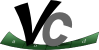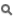Vc  1.1.0 SIMD Vector Classes for C++Introduction

Recent generations of CPUs, and GPUs in particular, require data-parallel codes for full efficiency. Data parallelism requires that the same sequence of operations is applied to different input data. CPUs and GPUs can thus reduce the necessary hardware for instruction decoding and scheduling in favor of more arithmetic and logic units, which execute the same instructions synchronously. On CPU architectures this is implemented via SIMD registers and instructions. A single SIMD register can store N values and a single SIMD instruction can execute N operations on those values. On GPU architectures N threads run in perfect sync, fed by a single instruction decoder/scheduler. Each thread has local memory and a given index to calculate the offsets in memory for loads and stores.

Current C++ compilers can do automatic transformation of scalar codes to SIMD instructions (auto-vectorization). However, the compiler must reconstruct an intrinsic property of the algorithm that was lost when the developer wrote a purely scalar implementation in C++. Consequently, C++ compilers cannot vectorize any given code to its most efficient data-parallel variant. Especially larger data-parallel loops, spanning over multiple functions or even translation units, will often not be transformed into efficient SIMD code.

The Vc library provides the missing link. Its types enable explicitly stating data-parallel operations on multiple values. The parallelism is therefore added via the type system. Competing approaches state the parallelism via new control structures and consequently new semantics inside the body of these control structures.

If you are new to vectorization please read this following part and make sure you understand it:

• The term vector used for data-parallel programming is not about the vectors you studied in math classes.
• Do not confuse vector with containers that also go by the same name. SIMD vectors actually do implement some aspect of a container, but they are closer to a fixed-sized std::array than to a dynamically resizable std::vector.
• The vector type in Vc is defined by the target hardware as a group of values with a fixed number of entries ( $$\mathcal{W}_\mathtt{T}$$). Typically one Vc::Vector object then fits into a SIMD register on the target system. Such a SIMD register consequently stores $$\mathcal{W}_\mathtt{T}$$ scalar values; in contrast to a general purpose register, which stores only one scalar value. This value $$\mathcal{W}_\mathtt{T}$$ is thus an unchangeable property of the hardware and not a variable in the Vc API. You can access the $$\mathcal{W}_\mathtt{T}$$ value via the static Vc::Vector::size() function. Since this function is a constant expression you can also use it for template arguments.
• Note that some hardware may use different vector register widths for different data types. For example, AVX has instructions for 256-bit floating point registers, but only 128-bit integer instructions, which is why the integral Vc::Vector types use the SSE implementation for AVX target systems.
Example 1:

You can modify a function to use vector types and thus implement a horizontal vectorization. The original scalar function could look like this:

void normalize(float &x, float &y, float &z)
{
const float d = std::sqrt(x * x + y * y + z * z);
x /= d;
y /= d;
z /= d;
}

To vectorize the normalize function with Vc, the types must be substituted by their Vc counterparts and math functions must use the Vc implementation (which is, per default, also imported into std namespace):

void normalize(float_v &x, float_v &y, float_v &z)
{
const float_v d = Vc::sqrt(x * x + y * y + z * z);
x /= d;
y /= d;
z /= d;
}

The latter function is able to normalize four 3D vectors when compiled for SSE in the same time the former function normalizes one 3D vector.

For completeness, note that you can optimize the division in the normalize function further:

const float_v d_inv = float_v::One() / Vc::sqrt(x * x + y * y + z * z);
const float_v d_inv = Vc::rsqrt(x * x + y * y + z * z); // less accurate, but faster

Then you can multiply x, y, and z with d_inv, which is considerably faster than three divisions.

As you can probably see, the new challenge with Vc is the use of good data-structures which support horizontal vectorization. Depending on your problem at hand this may become the main focus of design (it does not have to be, though).

Alignment

What is Alignment

If you do not know what alignment is, and why it is important, read on, otherwise skip to Tools. Normally the alignment of data is an implementation detail left to the compiler. Until C++11, the language did not even have any (official) means to query or modify alignment.

Most data types require more than one Byte for storage. Thus, even most atomic data types span several locations in memory. E.g. if you have a pointer to float, the address stored in this pointer just determines the first of four Bytes of the float. Naively, one could think that any address (which belongs to the process) can be used to store such a float. While this is true for some architectures, some architectures may terminate the process when a misaligned pointer is dereferenced. The natural alignment for atomic data types typically is the same as their size. Thus the address of a float object should always be a multiple of 4 Bytes.

Alignment becomes more important for SIMD data types.

1. There are different instructions to load/store aligned and unaligned vectors. The unaligned load/stores recently were greatly improved in x86 CPUs. Still, the rule of thumb says that aligned loads/stores are faster.
2. Access to an unaligned vector with an instruction that expects an aligned vector crashes the application. Once you write vectorized code you might want to make it a habit to check crashes for unaligned addresses.
3. Memory allocation on the heap will return addresses aligned to some system specific alignment rule. E.g. Linux 32bit aligns on 8 Bytes, while Linux 64bit aligns on 16 Bytes. Both alignments are not strict enough for AVX vectors. Worse, if you develop on Linux 64bit with SSE you won't notice any problems until you switch to a 32bit build or AVX.
4. Placement on the stack is determined at compile time and requires the compiler to know the alignment restrictions of the type.
5. The size of a cache line is just two or four times larger than the SIMD types (if not equal). Thus, if you load several vectors consecutively from memory every fourth, second, or even every load will have to be read from two different cache lines. This is called a cache line split. They lead to degraded performance, which becomes very noticeable for memory intensive code.

Tools

Vc provides several classes and functions to get alignment right.

• Vc::VectorAlignment is a compile time constant that equals the largest alignment restriction (in Bytes) for the selected target architecture.
• Vc::AlignedBase, Vc::VectorAlignedBase, and Vc::MemoryAlignedBase implement the alignment restrictions needed for aligned vector loads and stores. They set the alignment attribute and reimplement the new and delete operators, returning correctly aligned pointers to the heap.
• Vc::malloc and Vc::free are meant as replacements for malloc and free. They can be used to allocate any type of memory with an abstract alignment restriction: Vc::MallocAlignment. Note, that (like malloc) the memory is only allocated and not initialized. If you allocate memory for a type that has a constructor, use the placement new syntax to initialize the memory.
• Vc::Allocator is an STL compatible allocator class that behaves as specified in the C++ specification, implementing the optional support for over-aligned types. Therefore, memory addresses returned from this allocator will always be aligned to at least the constraints attached to the type T. STL containers will already default to Vc::Allocator for Vc::Vector<T>. For all other composite types you want to use, you can take the Vc_DECLARE_ALLOCATOR convenience macro to set is as default.
• Vc::Memory, Vc::Memory<V, Size, 0u>, Vc::Memory<V, 0u, 0u> The three different variants of the memory class can be used like a more convenient C-array. It supports two-dimensional statically sized arrays and one-dimensional statically and dynamically sized arrays. The memory can be accessed easily via aligned vectors, but also via unaligned vectors or gathers/scatters.